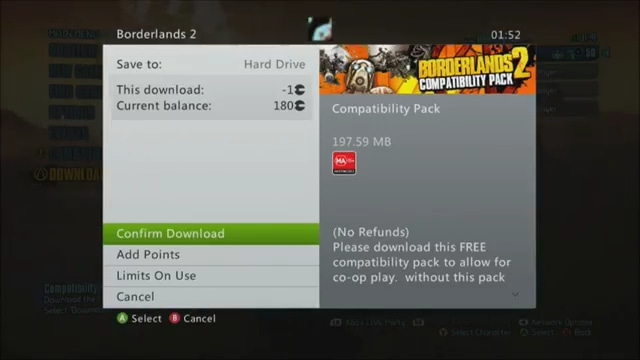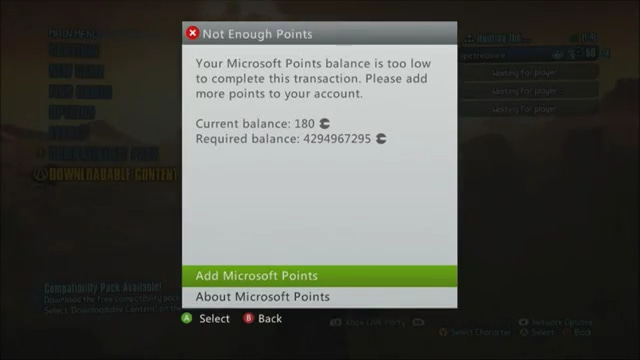# Borderlands 2 Compatibility Pack Pricing

by Tom Richards

Tuesday, October 09, 2012

Why did the Borderlands 2 compatibility pack cost -1 MSP, and then 4,294,967,295 MSP, simultaneously?

Well, somebody derped. Whether it was Gearbox or Microsoft, I’m not sure. Here are a few screencaps of the issue we’re talking about:This download costs -1 MSP.This download actually costs 4,294,967,295 MSP.

Here’s a short C code example of this problem.

``````#include <stdio.h>
int main()
{
int price = -1;

printf("Real price: %d\n", price);
printf("Derp price: %u\n", price);

return 0;
}
``````

The output of this program is:

``````Real price: -1
Derp price: 4294967295
``````

Wait, what? I didn’t type that crazy four billion number in my source code anywhere. So… what happened? Let’s dissect the code to figure it out.

``````int price = -1;
``````

First, we declare a signed integer variable with the name `price`, and the value of `-1`. Because it is a signed 32-bit integer, this variable is capable of holding any number between −2,147,483,648 and 2,147,483,647. -1 will fit in there just fine. The declaration of this variable produces no output.

``````printf("Real price: %d\n", price);
``````

We print out the price as a signed decimal integer, hoping all goes well. The `%d` part means “decimal integer”.

``````Real price: -1
``````

Looking good so far.

``````printf("Derp price: %u\n", price);
``````

Next, we tell the computer, “Hey! Go look at that price again but pretend like it’s an unsigned integer.” The `%u` part means “unsigned decimal integer”.

``````Derp price: 4294967295
``````

Oh no. This does not look right.

A 32-bit unsigned integer can represent values between 0 and 4,294,967,295. -1 is not in that range. When we look at a signed negative integer as though it were an unsigned integer, it will show up as a really large positive number. Usually it will be some number above 2,147,483,647.

I don’t know what all that fancy code stuff means. Why did -1 turn into 4,294,967,295?

To store negative numbers in a computer, we use something called the Two’s Complement representation of a number. If we want to convert a number into its Two’s Complement representation, we follow these steps:

1. Convert the number to binary as a positive number.
2. Flip all the bits (1 becomes 0, 0 becomes 1).
3. Add 1 to the number (in binary).

Let’s do this for -1.

1. Convert -1 to binary as a positive number.
`110 = 000000012`

2. Flip all the bits.
`111111102`

3. Add 1.
`111111112`

The computer would store -1 as `111111112` in a signed representation, assuming our numbers are only 8 bits.

Unfortunately, our value `111111112` was forcibly converted into an unsigned representation. Now it can now only be a positive number.

`111111112 = 25510`

In Microsoft Points world, however, numbers are represented with 32 bits. We can apply the same series of steps to find that the 32-bit representation of -1 becomes a really big number.

`111111111111111111111111111111112 = 4,294,967,29510`

#### tl;dr:

I guess I'm not playing Borderlands 2 anytime tonight.